# Posts Tagged ‘ Statistical Thinking ’

## On the assumptions (and misconceptions) of linear regression

August 27, 2018
By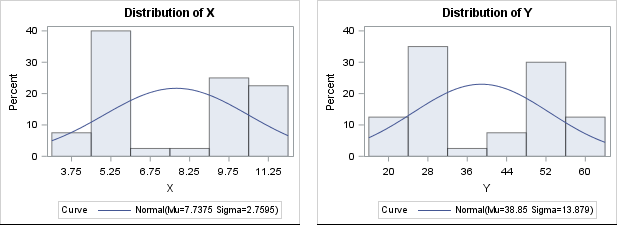A frequent topic on SAS discussion forums is how to check the assumptions of an ordinary least squares linear regression model. Some posts indicate misconceptions about the assumptions of linear regression. In particular, I see incorrect statements such as the following: Help! A histogram of my variables shows that they

Tags: , , ,
Posted in SAS | Comments Off on On the assumptions (and misconceptions) of linear regression

## Standardized regression coefficients

August 22, 2018
By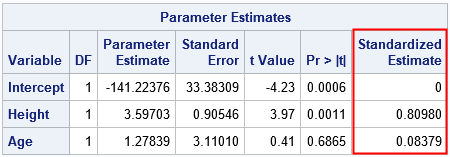A SAS programmer recently asked how to interpret the "standardized regression coefficients" as computed by the STB option on the MODEL statement in PROC REG and other SAS regression procedures. The SAS documentation for the STB option states, "a standardized regression coefficient is computed by dividing a parameter estimate by

Tags: , , ,
Posted in SAS | Comments Off on Standardized regression coefficients

## The probability that two random chords of a circle intersect

July 11, 2018
By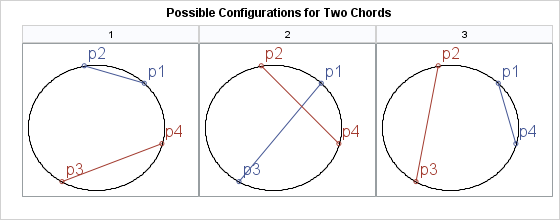In a previous article, I showed how to find the intersection (if it exists) between two line segments in the plane. There are some fun problems in probability theory that involve intersections of line segments. One is "What is the probability that two randomly chosen chords of a circle intersect?"

Tags: , ,
Posted in SAS | Comments Off on The probability that two random chords of a circle intersect

## Find the distance between observations and a target value

March 28, 2018
By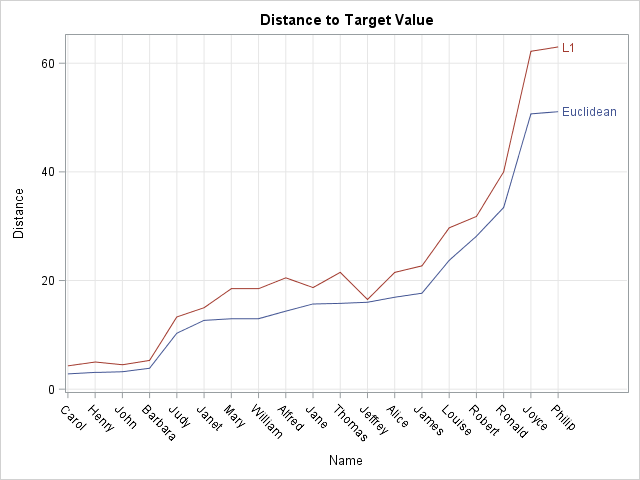Suppose you want to find observations in multivariate data that are closest to a numerical target value. For example, for the students in the Sashelp.Class data set, you might want to find the students whose (Age, Height, Weight) values are closest to the triplet (13, 62, 100). The way to

Tags: , ,
Posted in SAS | Comments Off on Find the distance between observations and a target value

## Data unavailable? Use the “eyeball distribution” to simulate

January 15, 2018
By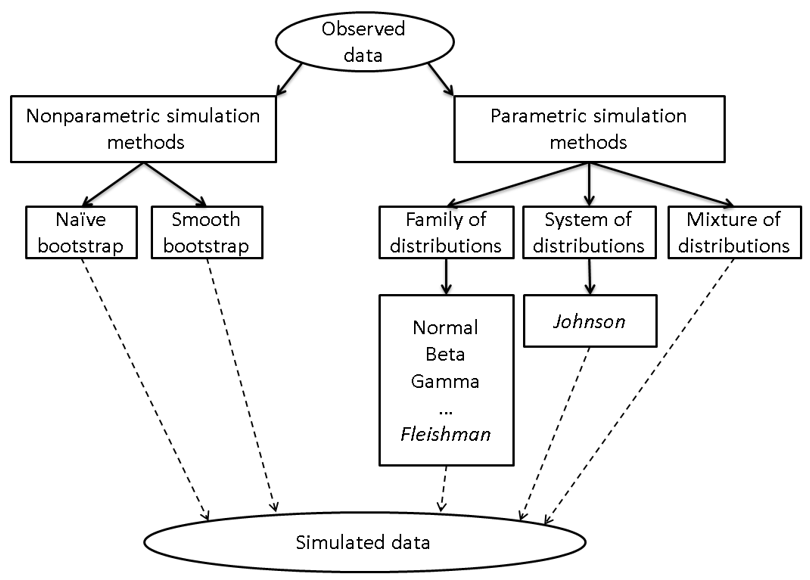Last week I got the following message: Dear Rick: How can I create a normal distribution within a specified range (min and max)? I need to simulate a normal distribution that fits within a specified range. I realize that a normal distribution is by definition infinite... Are there any alternatives,

Tags: , ,
Posted in SAS | Comments Off on Data unavailable? Use the “eyeball distribution” to simulate

## Should you use principal component regression?

October 25, 2017
By

This article describes the advantages and disadvantages of principal component regression (PCR). This article also presents alternative techniques to PCR. In a previous article, I showed how to compute a principal component regression in SAS. Recall that principal component regression is a technique for handling near collinearities among the regression

Tags: , ,
Posted in SAS | Comments Off on Should you use principal component regression?

## How to understand weight variables in statistical analyses

October 2, 2017
By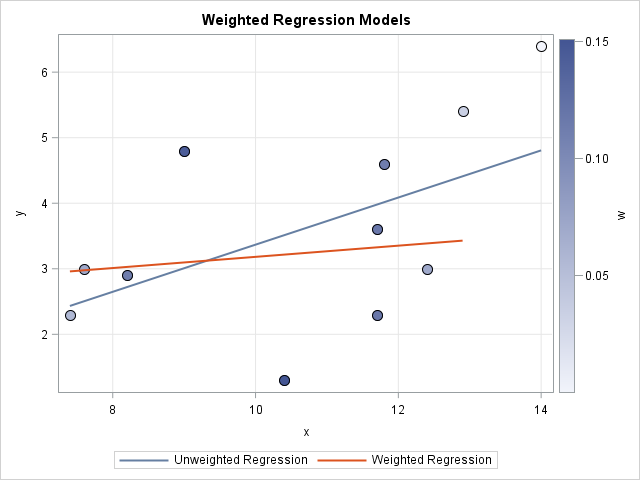How can you specify weights for a statistical analysis? Hmmm, that's a "weighty" question! Many people on discussion forums ask "What is a weight variable?" and "How do you choose a weight for each observation?" This article gives a brief overview of weight variables in statistics and includes examples of

Tags: , ,
Posted in SAS | Comments Off on How to understand weight variables in statistical analyses

## Fisher’s transformation of the correlation coefficient

September 20, 2017
By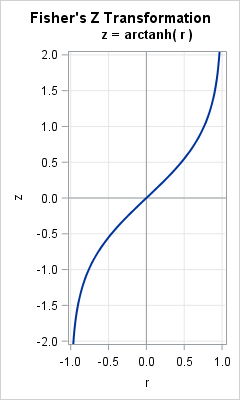Pearson's correlation measures the linear association between two variables. Because the correlation is bounded between , the sampling distribution for highly correlated variables is highly skewed. Even for bivariate normal data, the skewness makes it challenging to estimate confidence intervals for the correlation, to run one-sample hypothesis tests ("Is

Tags: , ,
Posted in SAS | Comments Off on Fisher’s transformation of the correlation coefficient

## Welcome!

SAS-X.com offers news and tutorials about the various SAS® software packages, contributed by bloggers. You are welcome to subscribe to e-mail updates, or add your SAS-blog to the site.Dear readers, proc-x is looking for sponsors who would be willing to support the site in exchange for banner ads in the right sidebar of the site. If you are interested, please e-mail me at: tal.galili@gmail.com